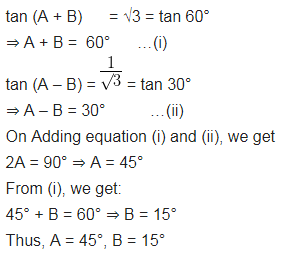# If tan (A + B) = √3 and tan (A – B)

If tan (A + B) = √3 and tan (A – B) =$\frac { 1 }{ \surd 3 }$; 0° < A + B ≤ 90°; A > B, find A and B.